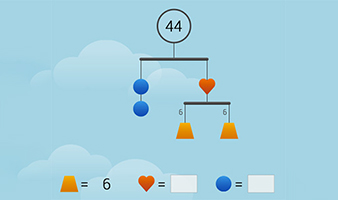Go to website

# SolveMe Mobiles

SolveMe Mobiles from the US National Science Foundation invites students to play with virtual mobiles: balanced structures with hidden values for the weights. These interactive puzzles help develop algebraic and problem-solving skills.

Year level(s) Year 2, Year 3, Year 4, Year 5, Year 6, Year 7
Audience Student
Format Interactive
Teaching strategies and pedagogical approaches Concrete Representational Abstract model
Keywords algebra, balance beam, unknowns

## Curriculum alignment

Strand and focus Algebra, Build understanding
Topics Algebraic expressions, Patterns and algebra
AC: Mathematics (V9.0) content descriptions
AC9M7A01
Recognise and use variables to represent everyday formulas algebraically and substitute values into formulas to determine an unknown

AC9M6A01
Recognise and use rules that generate visually growing patterns and number patterns involving rational numbers

AC9M5A02
Find unknown values in numerical equations involving multiplication and division using the properties of numbers and operations

AC9M4A01
Find unknown values in numerical equations involving addition and subtraction, using the properties of numbers and operations

AC9M3A01
Recognise and explain the connection between addition and subtraction as inverse operations, apply to partition numbers and find unknown values in number sentences

AC9M2A01
Recognise, describe and create additive patterns that increase or decrease by a constant amount, using numbers, shapes and objects, and identify missing elements in the pattern

Numeracy progression Number patterns and algebraic thinking (P3, P4, P5, P6)
Multiplicative strategies (P7)